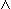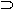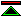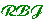# The Logical Foundations of Mathematics

### by William S. Hatcher

 Chapter 1 - First Order Logic A presentation of first order logic including a general treatment of "variable binding term operators", such as set comprehension, which are often required in foundation systems. Chapter 2 - The Origin of Modern Foundational Studies Chapter 3 - Frege's System and the Paradoxes Chapter 4 - The Theory of Types Chapter 5 - Zermelo Fraenkel Set Theory Chapter 6 - Hilbert's Program and Gödel's Incompleteness Theorems Chapter 7 - The Foundational Systems of W.V.Quine Chapter 8 - Categorical Algebra

## Chapter 2 - The Origin of Modern Foundational Studies

Section 1 - Mathematics as an Independent Science
Section 2 - The Arithmetisation of Analysis
Section 3 - Constructivism
Section 4 - Frege and the notion of a Formal System
Section 5 - Criteria of Foundations
"What must a foundation be, and what must it do?"

## Chapter 8 - Categorial Algebra

Introduction
In the introduction, Hatcher describes the relevance of category theory for foundations.
Section 8.1 The notion of a category
An informal introduction to category theory.
Section 8.2 The first order language of categories
A formal account in the form of the first order theory of categories.
Section 8.3 Category Theory and Set Theory
A look at problems arising in doing category theory on a set theoretic foundation.
Section 8.4 Functors and Large Categories
Now we learn about the distinction bewteen large and small categories, and of the kinds of constructions that fail to be closed in classical set theory.
Section 8.5 Formal Development of the Language and Theory CS
The axiomatisation of Categories, C, is now specialised to an axiomatisation of the category of sets, CS.
Section 8.6 Topos Theory
Topos theory provides an alternative approach to axiomatisation of the category of sets. This is generalised in the sense that the objects need not be classical sets.
Section 8.7 Global Elements in Toposes
Section 8.8 Image Factorisations and the Axiom of Choice
Section 8.9 A last look at CS
Section 8.10 ZF and WT
Section 8.11 The Internal Logic of Toposes
Section 8.12 The Internal Language of a Topos
Section 8.13 Conclusions

## Chapter 2 - The Origin of Modern Foundational Studies

### Ch2 Section 5 - Criteria of Foundations

The criteria Hatcher identifies are:

1. A foundation must be adequate for a reasonably large portion of mathematics
2. A foundation must derive from some intuitively natural principles
3. The basic principles and (undefined) notions should be as economical as possible.
4. The foundations must be consistent.
5. The foundation should be expressed (or expressible) as a formal system.

Of these the first might be considered parasitic on ones ideas of the extent of mathematics. However, Hatcher relates this issue to the Gödel incompleteness results, which concern how much can be proven in any area of mathematics, not which areas of mathematics can be addressed.

The second and third are reasonable but not essential requirements. No formal system can be used in practice without good tool support, and for this reason among others formal systems are rarely used by mathematicians in practice. However, even with current standards of tooling the distance between the primitive principles and those derived rules and principles used in practice is so great that the relevance of this criterion is not clear.

Two alternative criteria which may be more appropriate in the context of good mechanised support for developing mathematics formally are:

1. That the semantics of the system are intuitive.

Since semantic intuitions remain important even where detailed reasoning with the primitive rules is automated.

2. That the primitive rules are convenient for automated reasoning.

The fourth criterion is crucial, and the fifth a precondition of any high level of confidence in the fourth.

## Chapter 8 - Categorial Algebra

### Introduction

Hatcher describes the relevance of category theory for foundations as consisting in:
• Category theory "has raised interesting questions about the commonly presumed irreducible character of the notion of set and membership".

Because category theory has supplanted set theoretic reasoning in many areas of mathematics.

• There are many constructions commonly carried out in category theory that cannot be done in a natural way in most set theories. Or at all in ZF or NBG.

Hatcher is, at this point, rather coy about what these constructions are.

It seems a weak story. On the one hand Hatcher is imprecise about the nature of the issues which he claims category theory to be raising, and secondly, it is unclear how category theory is to provide a foundation system which improves our standing in relation to these issues. However, this is just the introduction, once we learn what a category is we will get a clearer view of the issues.

### Ch8 Section 2 - The first-order language of categories

 C.1. (x1)(D(C(x1)) = C(x1)(x1)(C(D(x1)) = D(x1) The domain of the codomain of x1 is the codomain of x1, and the codomain of the domain of x1 is the domain of x1. C.2. (x1)(x2)(x3)(x4) (K(x1, x2, x3)K(x1, x2, x4)x3 = x4 The composition of x1 with x2 is unique when it is defined. C.3. (x1)(x2)((Ex3) K(x1, x2, x3)(C(x1) = D(x2))) The composition of x1 with x2 is defined if and only if the codomain of x1 is the domain of x2. C.4. (x1)(x2)(x3)(K(x1, x2, x3)( D(x3) = D(x1)C(x3) = C(x2)) If x3 is the composition of x1 with x2 then the domain of x3 is the domain of x1 and the codomain of x3 is the codomain of x2. C.5. (x1)(K(D(x1),x1,x1)K(x1,C(x1),x1) For any x1, the domain of x1 is a left identity for x1 under composition and the codomain is a right identity. C.6. (x1)(x2)(x3)(x4)(x5)(x6)(x7)((K(x1, x2, x3)K(x2, x4, x5)K(x1, x5, x6)K(x3, x4, x7))( x6 = x7) Composition is associative when it is defined.

### Ch8 Section 3 - Category Theory and Set Theory

Many of the categories which we may wish to discuss represent in one way or another, a problem from the perspective of classical set theory. For example, consider the category of groups. For every set there is at least one group whose carrier is that group. The number of groups in set theory is therefore as large as the number of sets, and by the limitation of size principle implicit in most accounts of set theory the category of all groups will not therefore be a set.

In Zermelo set theory, there being nothing but sets, the category of all groups simply doesn't exist. The best we can get, by analogy with settling for formation of sets by separation rather than comprehension, is the category of all groups whose carrier is a subset of some given set. Alternatively, in a system such as NBG, while the category of all groups exists, it is a proper class. In general, where a set theory has its semantic basis in the iterative conception of set (excluding Quine's systems) a distinction of kind or size is likely to be necessary, and the formation of the category of groups up to a certain size will turn out to be larger.

"We feel that the category of groups, or the category of sets ought to be a fixed structure with a definite theory. In fact their structure varies according to the various foundational systems in which we may chose to work."

This contrasts with our intuitions about structures such as the natural and real number systems. In these cases, though we know from Gödel that the results provable vary according to the proof theoretic strength of the foundation system we adopt, we do not perceive of the foundation system as affecting the structure of these number systems. (actually Hatcher appears to believe that what is provable about these number systems does not vary between foundation systems.)

### Ch8 Section 4 - Functors and Large Categories

Now we start to look at the kinds of category theoretic construction which don't work out well in classical set theory.

If we choose some particular foundation system, say NBG, and try to work within the limits it imposes on the categories we can form, we then find that this collection of categories is not closed under the all the constructions that we are likely to want to perform on categories.

In this case we will find that categories fall into two kinds, those whose morphisms form a set (which are called small), and those whose morphisms are a proper class (and are called large categories).

Often it may be desired to form a category whose objects are themselves categories, and whose morphisms are functors between these categories. This will only be possible for collections of small categories.

Worse, even the functors between two categories will not form a class in NBG if the categories themselves are large.

"Our second foundational problem of categories can be stated as follows:Certain intuitive constructions on categories cannot be performed indiscriminately for large and small categories".

These and similar considerations lead one to consider "whether it might not be possible to found mathematics on category theory itself".

"At the very least" an analysis of set theory from the standpoint of category theory would lead to interesting new insights into its structure.

### Ch8 Section 13 - Conclusions

Until recently Hatcher says (in 1982), most mathematicians paid little attention to foundational issues, supposing that foundational problems had either been solved or were irrelevant.

The idea that there was a definitivei solution to foundational problems was however refuted by the independence results in ZF, which "tended to destroy set theory's (largely unwarranted) image as an absolute foundation for mathematics".

The idea that foundational issues are irrelevant is undermined by the number of problems in mathematics which turn out to be reducible to set-theoretic principles which are independent of the axioms of ZF.

Alongside this "loss of absolute faith in set theory" there has been "the rise of attractive alternatives such as category theory".

Hatcher then concludes that "what is truly foundational" is "certain key, unifying notions common to many different aspects of mathematical practice", e.g. "the notions of universality and naturality in category theory".

It is not clear how this kind of foundation meets the criteria for foundations enunciated by Hatcher in section 2.5, so we must presume that the influence of category theory has lead Hatcher to revise his criteria in ways which he does not make explicit.©created 1995/3/16 modified 1999/10/16